## Friday 30 October 2009

### Counting LinesMy lunchtime math problem solving group was going through some old American Mathematics Competition problems, and we came across one that asked, in slightly more complicated language, "How many lines can be drawn that intersect at least two points in a 3x3 rectangular array?".

Most of the kids who understood the wording got the answer, and when I asked them how, they said they counted... they drew the dots, drew the lines, and counted them...

So I asked, how would you do it if they had a ten-by-ten array?

Counting the number of lines in an nxn array that contain the maximum number of points, n, is pretty straightforward. There are n horizontal lines that contain n points, and another n vertical lines, and two diagonals. For nxn there are 2n+2 lines that contain n points. In the 3x3 case, there are 2(3)+2 = 8 lines that contain exactly three points. So how to count the 2x2.

After a while I decided that there would be a maximum of n choose 2 lines if no two were collinear, so at most, a set of nine points could contain 9 choose 2 = 36 lines. Now each of the lines that had three points, would use up three different lines from this 36.. think of a line with points A, B, and C all collinear. Instead of three lines, they fall on one, so each line of three points would reduce the possible total of 36 by two... and since there are 8 of these lines, we need to eliminate 8x2=16 lines from the 36 to get a total of 20, 8 with three points, 12 more with only two points.

This verified the students solution by counting, but didn't bring us much closer to the general ten x ten case. For instance in a 4x4 there are some lines with four points (10 of them) and some more with three points (a quick look assured me there would be four) and the rest with only two points. By Starting with 16 choose 2 and eliminating duplication as before, I figured out that there would be 48 of the lines with two points.

By the next time we meet, Conner S. had counted up the number of lines with exactly two points by hand up to n=5 (and had a small error in the n=5 case finding only 100 when there are 108... Examining the case for n=5 is influential, but still, we don't get a really good clue of a general pattern. The sequence for the number of total lines from n=2 to n=5 is 6, 20, 62, 140... you might want to try to find the next one.. I couldn't, and the pattern of lines with exactly two points was equally wild... 6, 12, 48, 108??? (For my students, I will show how the counting technique used above to the possible lines given by n choose 2 to find the number of lines with two points. In most cases (such as the five by five) it is not too hard to count the number of lines that contain three, four, or five points.)

I guess I can be ok with my kids not being able to find the pattern, as it seems that the pattern didn't have any simple solution at all, and was eventually extended, it seems, beyond the hand countable cases by computer search. Afterward they did find a summation notation that is way complicated. I finally searched the On-line integer data base and found the actual numbers... don't look if you are still figuring out the pattern for yourself. This triangular array gives the number of lines containing exactly k points in an nxn array.. At the top of the triangle is n=2, k=2, and as you go down each row the n goes up one... so the numbers in the fourth row(for n=5, a 5x5 array), {108, 16, 4, 12} is the number of lines containing 2, 3, 4, and 5 points.Interesting to look down the center at the sequence of 4's, as long as the number of points in the line is more than (n+1)/2 and less than n, there will be four such lines. Predicting the rest of the table as yet is beyond me.. if you see a simple way to calculate these values, do please advise.

## Saturday 24 October 2009

### A Cool Tool, Derivatives Without CalculusAfter the AP Calculus exam is over and there are still a few weeks of school left, I like to show my students some interesting things that are related to calculus ideas, but have no actual calculus in them. One of those ideas is finding derivatives of polynomials using synthetic division, and another is the idea of how the Polar of a point gives the tangent line to a point on a quadratic curve without calculus.

A brief explanation for those who are not aware of this method. a standard type of problem in calculus is to take a conic, such as an ellipse, and use implicit differentiation to find the tangent line to a point on the curve. For example we might takeand find the tangent at some point such as (3/sqrt(10),6/sqrt(10)). It is a nice example because most capable students can solve it for y and do the derivative of the positive half of the ellipse and find the derivative, but it is gets a little messy. Then they can do it by the implicit method and see that (assuming all was done correctly) they get the same result.. the calculus they are learning seems to agree with the stuff they already feel they know.

Now for the Polar method. It seems that if you take the equation, and replace one of the two variables in each square with the value of the point, you get the value of the tangent. For example, the tangent tois found by writing the equation. The image at top shows the ellipse and its tangent.

It seems a little harder to do with a parabola because one of the terms is not a square, but you can replace the y with (y+c)/2 (where c is the y-value at the point of tangency we seek. For example the tangent to the curve y=x2 at the point (2,4) is given by (y+4)/2 = 2x. When simplified to y=4x-4, most semi-capable calculus students will recognize that it is correct.

I was recently wondering about this idea. I had always thought it only applied to quadratics, and had never seen it applied beyond that level. I began to wonder what would happen if you applied it to a cubic, so I picked an easy one, y=x3 at the point (1,1)to see what would happen. Replacing y with (y+1)/2 and one x with 1, and another with (x+1)/2 we get (y+1)/2= (1)(x)(1+x)/2, which simplifies to y=x2 + x -1 . The two graphs are shown below.Ok, its tangent, but not a linear tangent... that was revealing in itself.. then it hit me that the tangent to y=x2 + x -1 at (1,1) has a slope of three, so if I applied the polar approach one more time, I would get the familiar tangent line we found in calculus...

I don't yet understand how these descending degree equations tangent to the same point are related, and plan to play with them some more... if you understand a little more than I what is happening here, and how it relates to the traditional calculus, a tutorial would be appreciated. I did follow up and check it out with x3 + 3xy + y2 = 4 and the same process works for a mixed implicit function (at least it did with this one).

Enough for now, but more ahead.

## Thursday 22 October 2009

### The Lucasian Chair, From the FFT of Calculus, to String Theory

This month the Lucasian Chair of mathematics at Cambridge, just down the road from my school here in Lakenheath, was passed to Michael Green, a physicist and pioneer in String Theory. Officially, I believe, he will replace the retiring Stephen Hawking on November first.

The Lucasian Chair began with a grant of land (and a huge library of books)from the Member of Parliament to the university, Henry Lucas, to provide a 100 pounds sterling annual stipend. The first chair was Issac Barrow, a classical mathematician. It may be surprising to young people who are taught that Newton invented the Calculus, that Barrow, Newton's teacher, gave the first general proof of the First Fundamental Theorem of Calculus, the one that links integration and differentiation together.
Amazingly, after only five years, he gave up the chair to his student, Newton. Newton is probably one of the two most famous holders of the chair, the next most well known being the retiring emeritus chair, Stephen Hawking.

After Newton, the chair passed through a succession of mathematicians that would not be recognizable to the common lay person. In fact, his successor, William Whiston, would probably not be a name recognized by most mathematicians. Born in 1667, the year after the great fire of London, he was headed for the top, and rubbing elbows with the best for a while. He served as Newton’s deputy at Cambridge, where he was a fellow. He was one of the earliest proponents of the theory that comets had a periodic behavior, along with Halley. He was a classical scholar and mathematician who, if remembered at all, is remembered for his contributions to recreational mathematics. His translation of the works of Flavius Josephus may have contained a version of the famous Josephus Problem, and in 1702 Whiston's Euclid discusses the classic problem of the Rope Round the Earth, (if one foot of additional length is added, how high will the rope be). I am not sure of the dimensions in Whiston's problem, and would welcome input, I have searched the book and can not find the problem in it, but David Singmaster has said it is there, and he is not a easy source to reject. It is said that Ludwig Wittgenstein was fascinated by the problem and used to pose it to students regularly.
Whiston was expelled from his chair on 30 October 1710; at the appeal of the heads of colleges. Comets were also part of this disaster in his life. He had become famous for his studies that stated that the Biblical flood had been caused by a comet, and gave support for other geological impacts of comets on the Earth. Whiston was removed from his position at Cambridge, and denied membership in the Royal Society for his “heretical” views. He took the “wrong” side in the battle between Arianism and the Trinitarian view, but his brilliance still made the public attend to his proclamations. When he predicted the end of the world by a collision with a comet in October 16th of 1736 the Archbishop of Canterbury had to issue a denial to calm the panic.

After two holders who seemed to be little more than groveling toadies, (Saunderson and Colsen) the chair passed to Edward Waring in 1760. In number theory, Waring's problem, proposed in 1770, asks whether for every k there exists a positive integer n such that every natural number is the sum of at most n kth powers of natural numbers (for example, every number is the sum of at most 4 squares), . The problem and its affirmative solution is now called the Hilbert–Waring theorem, proven by Hilbert in 1909.

I will continue this study anon...

## Tuesday 20 October 2009

### SuperProfessorDead tired and still couldn't sleep last night, so I thumbed through some old journal articles Dave Renfro sent me... and in the Spring 1978 Pi Mu Epsilon Journal they had one called "Superprof"...

Among the comparisons...
The Professor is stronger than a locomotive
The Associate Prof is stronger than a bull elephant
The Assistant Prof is stronger than a bull
The Instructor shoots the bull
The Grad Assistant smells like a bull....

The Prof. talks with God
The Associate prof talks with the angels
The Assistant Prof talks to himself
The instructor argues with himself
The Grad Asst loses arguments with himself..

and one that was sort of a math insider joke
The Prof. Creates consistent sets of axioms
The Associate Proves original theorems
The Assistant accepts axioms
The Instructor proves axioms

## Monday 19 October 2009

In a recent blog, I asked what I thought was An Interesting Geometry Problem. Several emails and a comment later, I thought it was time to give a solution.

Before I do, I want to gloat. I gave the problem to some of my students in seminar today, which includes a couple of bright young students, and in a matter of moments, Tyler P. and his Ti-89 solved it. He began by assuming "If it is true for any rectangle, it is true for a square." and worked from there. I don't know if that really makes the solution simpler, but it is the type of problem solving that marks a budding mathematician. He got the solution, but didn't recognize it as a special number, although when I told him what it was, he had heard of the name... note to self, give my students more exposure to recreational math topics.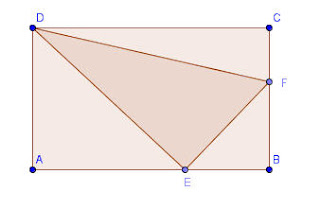So here is my solution, hope it is correct.
For ease of notation, I will refer to the lengths AE as x, EB as y, so that CD=x+y ... and similarly I will let BF=s and FC=t so that AD=s+t

Since the three triangles ADE, EBF, and FCD are all equal in area, it must be true that (s+t)x = ys=(x+y)t.

Expanding the first and last of these we get sx+tx=xt+yt and so sx=yt if we divide by xt we reveal the fact that s/t=y/x and so we see that the ratios of the partitions are equal on each side with corresponding parts adjacent to vertex B.

If instead, we divide sx=yt by only the t we have y=sx/t.

Now using that, we can substitute into the equality (s+t)x= ys to get sx + tx = s^2 x/t.

Factoring out an x, since it can not be zero, we get s + t = s^2/t and now if we divide all tems by t we get a quadratic in the ratio (s/t)... s/t + 1 = (s/t)^2 but this is quickly recognized to yield the conjugate of the divine proportion, ...Solving by any quadratic method we get s/t =so we see that the three triangles are all equal when the lengths AB and BC are divided in the golden ratio with the larger section adjacent to B.

If we find the area of one of these triangles, and compare it to the area of DEF, we derive a, perhaps unexpected, result that it is impossible to inscribe a triangle in a rectangle and have all four triangles have equal area.

Interestingly, the ratio of the area of DEF to the other triangles isor appx 2.23606797

## Saturday 17 October 2009

I wrote recently about a trigonometric version of Heron's Formula, and most folks who have played with geometry beyond the basics of high school are probably aware of an extension called Brahmagupta's Formula,; where s is half the perimeter, s=which gives the area of a cyclic quadrilateral (one whose four vertices lie on a common circle.

I was just reading a journal article from the American Mathematical Monthly from 1922 that Brahmagupta was not aware that the formula was only true for cyclic figures. It was not until Bhaskara in the 12th century that this concept was proven.

In the same article it points out that if you have four distinct lengths for the four sides, they can be rearranged to make three different inscribable quadrilaterals, but all three will have the same area.In addition, although there are six different diagonals, but they only have three different lengths. In the image, the diagonals that have a common color are the same length. The lengths of the diagonals can be found by another interesting relationship. There are three ways to form a sum of the products of two of the legs, ab+cd, ac+bd, and ad+bc. These three values can be combined to make the lengths of the diagonals. In the first of the figures above (order abcd) a and c are the opposite sides, so the two diagonals will be given by :Note that the diagonal that goes from the intersection of sides a and b to the vertex where sides c and d meet, is given when ab+cd is in the numerator, and similarly for the other diagonal.

But what about the circle itself, how might we determine the radius of a circle that contains an inscribable quadrilateral with area A. Use these same three ratios all multiplied together, :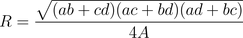If you treat a triangle as a degenerate cyclic quadrilateral (one side equals zero) then the last formula reduces to a somewhat well known formula for triangles, the circumcircle of a triangle is given by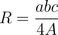## Friday 16 October 2009

### National Statistics DayThis Sunday (Oct 18, 2009)is National Statistics Day in Japan. (I am reminded that I heard a few years ago from Ron Dirkse, who used to teach at the American School in Japan, that the Japanese word for statistics, toukei, is pronounced much like the last name of the great statistician, John Tukey. Ron added that "according to a native speaker the tou means something like 'put together' and the kei is 'measure, calculate or total'. She thought it was interesting that there was a Tukey famous in statistics, but this word pre-dates him by a lot.")

It seems many countries in the world have such a celebration. It seems to be coordinated by the UN, and their web site says, "One hundred one countries and areas have been celebrating a statistics day, week or month to raise the profile of official statistics. There are variations in the way these events are held. About 80 of the countries celebrate a statistics day annually or intend to do so in the future. Some countries observe either a week-long event, or celebrate a statistics day every 5 years or did so as a one-time occasion."

Missing from the list??? Everyone in North America, the UK, France, Italy, China and Russia; among the large countries, but present of note is Australia, Germany, and a united approach with most of Africa celebrating on November 18, this year.Some seem to have been doing this for a long time...In Poland, it seems, they have been celebrating a statistics day for 90 years...

## Thursday 15 October 2009

### Higher Powers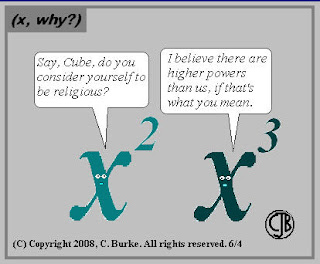Just came across a really interesting math cartoon site called (x, why?) , including the one above. And I am giving the one below to my calc class tomorrow morning...and then wait.... as my young nephew Zack says after every joke..."Do ya' get it?"And for stats class; I came across this one a few days ago but forgot where,... but think it is from Brown Sharpie...## Tuesday 13 October 2009

### An Interesting Geometry ProblemCame across a geometry problem with an interesting result which I did not suspect... thought I would share...
In the figure, rectangle ABCD is inscribed with a triangle by selecting points E and F on the segments AB and BC respectively, so that triangles AED, BEF, and CFD all have equal area. Find the ratios of AE:EB and BF:FC. I was surprised, and pleased, hope you are as well.

## Monday 12 October 2009

### You Might Be a Mathematician IF...

I always loved those Jeff Foxworthy "You might be a red-neck" routines, even when they struck a little too close to my Arkansas roots... So when I came across a Fall 1998 article in The Pentagon, with the same title as this blog, I wondered if it would be funny... I think it may be to non-mathematicians (maybe true red-necks don't think Jeff Foxworthy is funny, either) but it does earmark some of the ways we are different.

There were nineteen items in the list (to which I might add, you might be a mathematician if you count how many items are in long lists).. Here are a few of them, and some reflections.

You might be a mathematician if...

... you have constructed a regular 17-gon with straight-edge and compass (not guilty, but I have done a regular pentagon)

... you videotaped NOVA's presentation of Fermat's Last Theorem and watched it more than once.... (GUILTY.. I even have shown it to my classes... and worse, I also showed them Fermat's Last Tango)

... you've ever tried to prove Fermat's Last Theorem (guilty, repeatedly... dreamed I had the solution and woke up to find.... oooppps, what was that elegant last step)

.... you have ever spent more than two days working on the same problem (guilty, repeatedly, but I'm pretty slow)

... You know whether your birthdate (mmddyy) is a prime (Guilty, and I even know the prime factorization, 22x17x1483; Ok, the truth is, I even did that with the mmddyyyy to get 23 x 32 x 31 x 4517.... but I am not a complete nerd, I never did it the British way ddmmyy...

... you have ever extracted a cube root by paper and pencil..(guilty, but I didn't really enjoy it... well, only a little)

.... you can't tell the difference between a doughnut and a coffee cup...(actually I can, and I know what kind of coffee cup the joke refers to ... see my blog here ...

....you know who Paul Erdos was...(to which I add, you know how to pronounce it... substitute Euler for the same test.. and my ex-students all know I just crossed myself)

.... YOu know the prime factorization of your social security number (not guilty when I first read it, but then couldn't resist factoring it out, won't give the whole thing, but it does have a seven digit prime... which is pretty cool)

.... you've ever picked Pi when asked to pick a number between one and ten (guilty, so often that now my students are more likely to guess pi or e than they are an integer)

..... you ever tried to calculate the number of blades of grass in your lawn.(too easy, my brown grass is so sparse it is easy to count, but I did estimate the number of grains of salt in a pound of salt [off by about 5%])

...you've ever looked at a word problem and said, "Cool!" (not for a problem, but I have seen some solutions that made me say that, and more... Joshua Zucker's proof of the 25 stones problem is an example)

There were a couple of others, but some additional ones that seem to be appropirate :
... you try to mentally facto license plate numbers, or the time on a digital clock.

....you know how to figure out the day of the week people were born on when you hear their date of birth.

... you can't pass up a list like this without sending in an additional item... so go ahead, send it... if you are mathematician enough....

## Monday 5 October 2009

### The Absence of Applied GeometryI'm back in the USA this week on emergency leave. My father-in-law is terminally ill and the family is gathering to try to send him off in a way that is appropriate to his status as an Irish father of eleven and a great guy. Along the way I had the chance to be reacquainted with one of my biggest pet peeves, people who haven't learned enough geometry to stand at a baggage carousel. Ok, that seems like almost everyone... maybe math professors are even doing it.

Even before the thing starts to move, they jockey for position and stand as close as they can to the edge, then when it starts to move, they realize they can only see about five feet, so they lean over, one by one, to farther obscure each others view until they can only see about two feet.... but if they all stepped back about three feet, they could see from end to end, know when their bag was coming... and step up and grab it and go... It seems too obvious ... they are so bad at applying simple geometry, you expect them to walk a zig-zag path to customs because they don't intuitively know the shortest route.... Oh well, So long Gil, We love you guy.

## Friday 2 October 2009

### A Limit Approach to a Classic Geometric Probability Problem

One of the common problems from probability texts is : "A stick is broken into three parts. What is the chance that the sum of the lengths of eveiy two is greater than the length of the third that is that the three parts will form a triangle?"

The traditional method for an area approach for pre-calculus students is to begin by assuming the stick is of unit length, and the breaks occur at distances of x, and x+y from one end, giving three pieces of length x, y, and 1-x-y. Then a graph of all possible outcomes can be found by graphing the lenghts x and y on the coordinate plane. The regiong bound by x>0, y>0 and x+y<1 defines a triangle containing all possible solutions. To find the subset which will fit together to form a triangle, we think about the basic triangle inequality from plane geometry; "any two sides must be greater than the third side." That restriction means x <1/2, (otherwise, the other two sides will be less than x) and similarly y<1/2. Finally, since the remaining part, 1-x-y must also be less than 1/2, x+y > 1/2. By adding these three lines to our graph, we see that solutions which make a triangle form must fall into the smaller triangle, which is 1/4 of the total sample space of outcomes.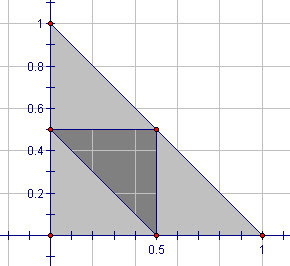I was reminded of this problem by an article in the Mathematical Spectrum, Vol 2, #2. by L. Rade. He begins with a finite version of the problem. A stick of length five units is cut into five equal spaces with six endpoints at A0 to A5.

Two of the four interior points are selected at random and the stick is broken at those two points. What is the probability that the broken pieces form a triangle?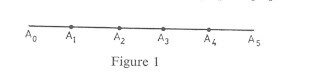He shows that there are $\dbinom{4}{2}$ , or 6 ways to break the stick, and only three of those will form a triangle. He then uses the lengths of the first two sticks (from the left) to plot a point on the xy plane to show the sample space of all solutions, and then a subset of those that form a triangle.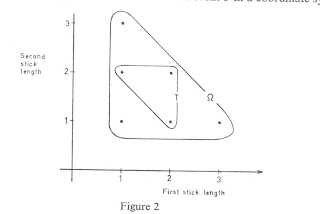Then he proceeds to show that for five, six, seven, and eight points equally spaced on the stick, the probabilities of getting a triangle are 1/2, 1/10, 2/5, and 1/7 respectively. Finally he generalizes the previous graph to n pointsand shows that the total number of points in the sample space is the triangular number for n-2, $\sum_{i=1}^{n-2}i = \frac{n^2-1}{8}$ .
Then he shows that the number of points in the sample space is given by a different formula if n is even or odd, and produces the probability of forming a triangle as[and guess who is doing piece-wise functions right now in pre=calc]
Finally, we derive the answer for an infinite number of possible points by letting n go to infinity and examine the graph of P(n)I think this might be a very effective way to present this problem, and probably end up with a little less of the glassy eyed stare when you finish; and it might also provide a good model for them to attack geomtric probability problems in the future.

A history note:
Dr. David Singmaster's web page on the chronology of recreational mathematics gives "1873 Lemoine considers Probability that Three Lengths Form a Triangle." However, I have found the problem presented in the 1865 "A treatise on infinitesimal calculus" by Bartholomew Price. . I have asked Dr. Singmaster to comment, and if he has additional detail, I will include it here in a future edit.
and a followup question Given that the broken stick forms a rectangle, what is the probability that the triangle is acute? I just noticed after I had typed all this, that the followup question was sent to the Mathematical Spectrum by the same Dr. David Singmaster I have referred to above.

And a followup history note: J. J. Sylvester was the first to pose this question in 1865, according to David Singmaster's chronology.

just ... fiddling around.. , this is me trying to write piecewise functions in LaTeX
\$f(x)=\begin{cases} \frac{n-4}{4(n-1}&\text{for n even } \\ \frac{n+1}{4(n-2)}&\text{for n odd} \end{cases} \$

## Thursday 1 October 2009

### Heron's Formula, The Trigonometric VersionHere is a nice problem to challenge your geometry teacher with. Ask her/him to find the volume of a parallelepiped (think of a box except the sides are not right angles... a prism with six faces, all parallelograms.) Probably they will say, "No problem, I'll find the base area and multiply by the height." Or maybe he is a vector fan and would prefer to use the scaler triple product. But which of those do you try if you are given the lengths of three non-parallel edges meeting at a vertex, call them x, y, and z, and the three angles between them which I will call a, b, and cs (for a reason that will be apparent later). The values don't matter, so make them up on your own.

I had my attention brought to this problem by an old journal article I was reading. The method caught my eye when I noticed that embedded in the formula was a trig looking version of Heron's (some say Hero's) formula for the area of a triangle. In the 1st century of the common era, Heron wrote a formula for the area of a triangle in his metrica. The formula, often appearing in High School texts, involves the semi perimeter of the triangle, s=$\frac{a+b+c}{2}$ where the lengths of the sides are a, b, and c. The area is given by $\sqrt{s(s-a)(s-b)(s-c)}$. The method was extended by Brahmagupta in the 7th century to give the area of any cyclic quadrilateral (one that is inscribed in a circle).

But for our case, it shows up as a relationship about the angles of the parallelepiped. For a parallelapiped with edges of length x, y, and z; which meet at mutual angles of a, b, and c, let s=$\frac{a+b+c}{2}$ as before, except now we are adding the angles. The volume is given by $2xyz\sqrt{sin(s)sin(s-a)sin(s-b)sin(s-c)}$. I kind of love when a "look-alike" formula pops up.

It reminds me of one more that is not well known to modern high school teachers. There is another variation of the law of sines for triangles in a plane $\frac{a}{sin(A)}=\frac{b}{Sin(B)}=\frac{c}{Sin(C)}$ (I really hate when that is written upside down).

It has a look-alike that works for spherical triangles; $\frac{sin(a)}{sin(A)}=\frac{sin(b)}{Sin(B)}=\frac{sin(c)}{Sin(C)}$. The spherical law of sines was first presented by Johann Muller, also known as Regiomontus,in his De Triangulis Omnimodis in 1464. This was the first book devoted wholly to trigonometry (a word not then invented). David E. Smith suggests that the theorem was Muller's invention.